Search

Inverse Functions: Definition of "Inverse" /
Drawing the Inverse from a Graph
(page 1 of 7)

Sections: Definition / Inverting a graph, Is the inverse a function?, Finding inverses, Proving inversesYour textbook's coverage of inverse functions probably came in two parts. The first part had lots of curly-braces and lists of points; the second part has lots of "y=" or "f(x)=" functions that you have to find the inverses for, if possible. The first part will show up in your homework and maybe on a test; the second part will definitely show up on your test, and you might even use it in later classes.

The inverse of a function has all the same points as the original function, except that the x's and y's have been reversed. This is what they were trying to explain with their sets of points. For instance, supposing your function is made up of these points: { (1, 0), (3, 5), (0, 4) }. Then the inverse is given by this set of point: { (0, 1), (5, 3), (4, 0) }. (Note that the order of the points doesn't matter; you can rearrange the points so the x's are "in order", or not. It's your choice.)

Once you've found the inverse of a function, the question then becomes: "Is this inverse also a function?" Using the set of points from above, the function above graphs like this: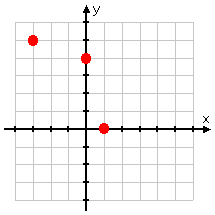You know that this is a function (and you can check quickly by using the Vertical Line Test): you don't have two different points that share the same x-value. The inverse graph is the blue dots below: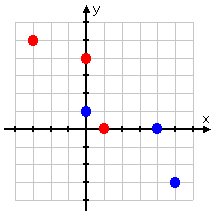Since the blue dots (the points of the inverse) don't have any two points sharing an x-value, this inverse is also a function.

Finding the inverse from a graph

Your textbook probably went on at length about how the inverse is "a reflection in the line y = x". What it was trying to say was that you could take your function, draw the line y = x (which is the bottom-left to top-right diagonal), put a two-sided mirror on this line, and you could "see" the inverse reflected in the mirror. Practically speaking, this "reflection" property can help you draw the inverse:

 Draw the points and the reflection line: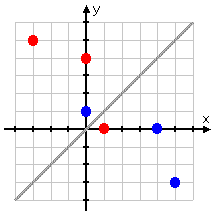Reflect the points across the line: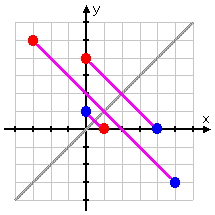You can see on this last picture that there is a definite graphical relationship between the points of the function and the points of the inverse. You can use this relationship if you're given a random graph and are told to graph the inverse.  Copyright © Elizabeth Stapel 2000-2011 All Rights Reserved

 Suppose you are given this graph: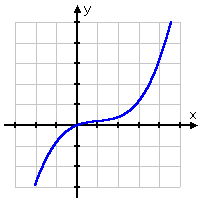Note that I have NOT told you what the function is!    Now draw the reflection line: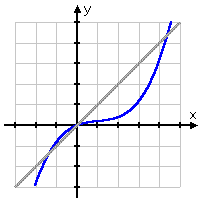(It would be a good idea to use a ruler for this; you'll want to be neat!).    Now eyeball the graph, and draw the diagonals from known points on the graph to their "reflections" on the other side of the line: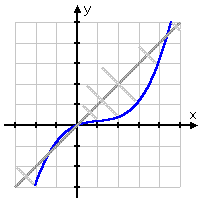Note that the points actually ON the line y = x don't move; that is, where the function crosses the diagonal, the inverse will cross, too.    Now draw in some plot-points: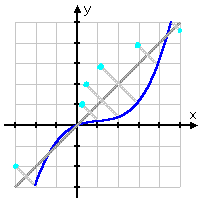...and connect the dots: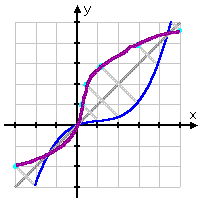Without ever knowing what the function was, you can draw the inverse (the purple line).

Top  |  1 | 2 | 3 | 4 | 5 | 6 | 7  |  Return to Index  Next >>

 Cite this article as: Stapel, Elizabeth. "Inverse Functions: Definition of 'Inverse' / Drawing the Inverse From a Graph."     Purplemath. Available from https://www.purplemath.com/modules/invrsfcn.htm.     Accessed [Date] [Month] 2016

Study Skills Survey

Tutoring from Purplemath
Find a local math tutor